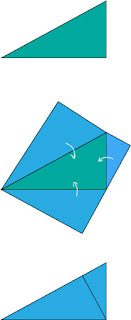### Pythagorean Pancakes

What do you like on your pancakes? Math? Good.

I like the fact that you can swap out the squares in your traditional Pythagorean recipe for other similar shapes:(here the areas of the two small hexagons are equal to the area of the biggie).
And if you swap them out for copies of the original right triangle, you get a beautiful "proof without words" of the Pythagorean Theorem:There are hundreds of proofs of the Pythagorean Theorem (if a triangle is right, then a² + b² = c²), but the pancake demo ask us to believe the converse (if a² + b² = c², then the triangle is right).  You can prove the converse several ways, but most of them use the Pythagorean Theorem as a step in the proof (if you find one that doesn't, let me know).